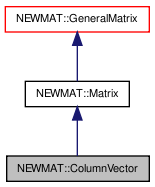# NEWMAT::ColumnVector Class Reference

`#include <newmat.h>`

Inheritance diagram for NEWMAT::ColumnVector:[legend]

## Detailed Description

Definition at line 896 of file newmat.h.

List of all members.

## Public Member Functions

ColumnVector ()
~ColumnVector ()
ColumnVector (ArrayLengthSpecifier n)
ColumnVector (const BaseMatrix &)
ColumnVector (const ColumnVector &gm)
void operator= (const BaseMatrix &)
ColumnVectoroperator= (Real f)
ColumnVectoroperator= (const ColumnVector &m)
Real & operator() (int)
Real & element (int)
Real operator() (int) const
Real element (int) const
MatrixType Type () const
GeneralMatrixTranspose (TransposedMatrix *, MatrixType)
void ReSize (int)
void ReSize (int, int)
void ReSize (const GeneralMatrix &A)
Real * nric () const
void CleanUp ()
void MiniCleanUp ()
void operator+= (const Matrix &M)
void operator-= (const Matrix &M)
void operator+= (Real f)
void operator-= (Real f)
void swap (ColumnVector &gm)

## Private Member Functions

GeneralMatrixImage () const

## Constructor & Destructor Documentation

 NEWMAT::ColumnVector::ColumnVector ( )

Definition at line 900 of file newmat.h.

Referenced by Image().

 NEWMAT::ColumnVector::~ColumnVector ( )

Definition at line 901 of file newmat.h.

 NEWMAT::ColumnVector::ColumnVector ( ArrayLengthSpecifier n )

Definition at line 902 of file newmat.h.

 NEWMAT::ColumnVector::ColumnVector ( const BaseMatrix & M )

Definition at line 111 of file newmat4.cpp.

 NEWMAT::ColumnVector::ColumnVector ( const ColumnVector & gm )

Definition at line 904 of file newmat.h.

## Member Function Documentation

 void NEWMAT::ColumnVector::CleanUp ( ) ` [virtual]`

Reimplemented from NEWMAT::GeneralMatrix.

Definition at line 908 of file newmat4.cpp.

 Real NEWMAT::ColumnVector::element ( int m ) const

Definition at line 762 of file newmat6.cpp.

 Real & NEWMAT::ColumnVector::element ( int m )

Definition at line 755 of file newmat6.cpp.

 GeneralMatrix * NEWMAT::ColumnVector::Image ( ) const` [private, virtual]`

Reimplemented from NEWMAT::Matrix.

Definition at line 802 of file newmat4.cpp.

 void NEWMAT::ColumnVector::MiniCleanUp ( ) ` [virtual]`

Reimplemented from NEWMAT::GeneralMatrix.

Definition at line 927 of file newmat.h.

 Real* NEWMAT::ColumnVector::nric ( ) const

Definition at line 924 of file newmat.h.

 Real NEWMAT::ColumnVector::operator() ( int m ) const

Definition at line 190 of file newmat6.cpp.

 Real & NEWMAT::ColumnVector::operator() ( int m )

Definition at line 78 of file newmat6.cpp.

 void NEWMAT::ColumnVector::operator+= ( Real f )

Reimplemented from NEWMAT::Matrix.

Definition at line 932 of file newmat.h.

 void NEWMAT::ColumnVector::operator+= ( const Matrix & M )

Reimplemented from NEWMAT::Matrix.

Definition at line 930 of file newmat.h.

 void NEWMAT::ColumnVector::operator-= ( Real f )

Reimplemented from NEWMAT::Matrix.

Definition at line 933 of file newmat.h.

 void NEWMAT::ColumnVector::operator-= ( const Matrix & M )

Reimplemented from NEWMAT::Matrix.

Definition at line 931 of file newmat.h.

 ColumnVector& NEWMAT::ColumnVector::operator= ( const ColumnVector & m )

Definition at line 907 of file newmat.h.

 ColumnVector& NEWMAT::ColumnVector::operator= ( Real f )

Reimplemented from NEWMAT::Matrix.

Definition at line 906 of file newmat.h.

 void NEWMAT::ColumnVector::operator= ( const BaseMatrix & X )

Reimplemented from NEWMAT::Matrix.

Definition at line 378 of file newmat6.cpp.

Referenced by operator=().

 void NEWMAT::ColumnVector::ReSize ( const GeneralMatrix & A ) ` [virtual]`

Reimplemented from NEWMAT::Matrix.

Definition at line 314 of file newmat4.cpp.

 void NEWMAT::ColumnVector::ReSize ( int nr, int nc ) ` [virtual]`

Reimplemented from NEWMAT::Matrix.

Definition at line 285 of file newmat4.cpp.

 void NEWMAT::ColumnVector::ReSize ( int nr )

Definition at line 275 of file newmat4.cpp.

Referenced by ReSize().

 void NEWMAT::ColumnVector::swap ( ColumnVector & gm )

Definition at line 934 of file newmat.h.

Referenced by NEWMAT::swap().

 GeneralMatrix * NEWMAT::ColumnVector::Transpose ( TransposedMatrix * , MatrixType mt ) ` [virtual]`

Reimplemented from NEWMAT::GeneralMatrix.

Definition at line 60 of file newmat5.cpp.

 MatrixType NEWMAT::ColumnVector::Type ( ) const` [virtual]`

Reimplemented from NEWMAT::Matrix.

Definition at line 433 of file newmat4.cpp.

The documentation for this class was generated from the following files:

 newmat11b Generated Mon May 9 04:54:19 2016 by Doxygen 1.6.3